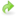# Verification¶

## Taylor remainder convergence test¶

The fundamental tool used in verification of gradients is the Taylor remainder convergence test. Let $$\widehat{J}(m)$$ be the functional, considered as a pure function of the parameter of interest, let $$\nabla \widehat{J}$$ be its gradient, and let $$\delta m$$ be a perturbation to $$m$$. This test is based on the observation that

$\left|\widehat{J}(m + h\delta m) - \widehat{J}(m)\right| \rightarrow 0 \quad \mathrm{at} \ O(h),$

but that

$\left|\widehat{J}(m + h\delta m) - \widehat{J}(m) - h\nabla \widehat{J} \cdot \delta m\right| \rightarrow 0 \quad \mathrm{at} \ O(h^2),$

by Taylor’s theorem. The quantity $$\left|\widehat{J}(m + h\delta m) - \widehat{J}(m)\right|$$ is called the first-order Taylor remainder (because it’s supposed to be first-order), and the quantity $$\left|\widehat{J}(m + h\delta m) - \widehat{J}(m) - h\nabla \widehat{J} \cdot \delta m\right|$$ is called the second-order Taylor remainder.

Suppose someone gives you two functions $$\widehat{J}$$ and $$d\widehat{J}$$, and claims that $$d\widehat{J}$$ is the gradient of $$\widehat{J}$$. Then their claim can be rigorously verified by computing the second-order Taylor remainder for some choice of $$h$$ and $$\delta m$$, then repeatedly halving $$h$$ and checking that the result decreases by a factor of 4.

In the case of PDE-constrained optimisation, computing $$\widehat{J}(m)$$ involves solving the PDE for that choice of $$m$$ to compute the solution $$u$$, and then evaluating the functional $$J$$. The main function in dolfin-adjoint for applying the Taylor remainder convergence test is taylor_test. To see how this works, let us again consider our example with Burgers’ equation:

from fenics import *

n = 30
mesh = UnitSquareMesh(n, n)
V = VectorFunctionSpace(mesh, "CG", 2)

u = project(Expression(("sin(2*pi*x)", "cos(2*pi*x)"), degree=2),  V)

u_next = Function(V)
v = TestFunction(V)

nu = Constant(0.0001)

timestep = Constant(0.01)

F = (inner((u_next - u)/timestep, v)

bc = DirichletBC(V, (0.0, 0.0), "on_boundary")

t = 0.0
end = 0.1
while (t <= end):
solve(F == 0, u_next, bc)
u.assign(u_next)
t += float(timestep)

J = assemble(inner(u, u)*dx)


As you can see, we here find the gradient only with respect to nu. Now let’s see how to use taylor_test: Instead of

dJdnu = compute_gradient(J, Control(nu))


we write

h = Constant(0.0001)
Jhat = ReducedFunctional(J, Control(nu))
conv_rate = taylor_test(Jhat, nu, h)


Here, h is the direction of perturbation. h must be the same type as what we are differentiating with respect to, so in this case since nu is a Constant h must also be a Constant. It is also a good idea to make sure that h is the same order of magnitude as nu, so that the perturbations are not too large. Jhat is the functional reduced to a pure function of nu, it is a ReducedFunctional object. We could also have taken the taylor test on the gradient with respect to the Function u. In that case h must also be a Function.

h = Function(V)
h.vector()[:] = 0.1
conv_rate = taylor_test(ReducedFunctional(J, control), u, h)


where control is defined as

control = Control(u)


At the desired time in the code. Here is the full program to check that we compute dJdnu correctly:

from fenics import *

n = 30
mesh = UnitSquareMesh(n, n)
V = VectorFunctionSpace(mesh, "CG", 2)

u = project(Expression(("sin(2*pi*x)", "cos(2*pi*x)"), degree=2),  V)

u_next = Function(V)
v = TestFunction(V)

nu = Constant(0.0001)

timestep = Constant(0.01)

F = (inner((u_next - u)/timestep, v)

bc = DirichletBC(V, (0.0, 0.0), "on_boundary")

t = 0.0
end = 0.1
while (t <= end):
solve(F == 0, u_next, bc)
u.assign(u_next)
t += float(timestep)

J = assemble(inner(u, u)*dx)

h = Constant(0.0001)  # the direction of the perturbation
Jhat = ReducedFunctional(J, Control(nu))  # the functional as a pure function of nu
conv_rate = taylor_test(Jhat, nu, h)Download the adjoint code with verification.

Running this program yields the following output:

$python tutorial4.py ... Computed residuals: [8.7896393952526051e-07, 2.2008124772799524e-07, 5.5062930799269294e-08, 1.3771065357994394e-08] Computed convergence rates: [1.9977677544105585, 1.9988829175084986, 1.9994412277283045]  The first line gives the values computed for the second-order Taylor remainder. As you can see, each value is approximately one quarter of the previous one. The second line gives the convergence orders of the second-order Taylor remainder: if the gradient has been computed correctly these numbers should be 2. As we can see they are in fact very close to 2, so we are calculating the gradient correctly. If you want to see if some object is the gradient you can pass the inner product of that object and the direction h with the named argument dJdm. For example we may want to check that the convergence orders of the first-order Taylor remainder are 1. This is achieved by passing a proposed gradient 0: conv_rate = taylor_test(Jhat, Constant(nu), h, dJdm = 0)  Adding this we get the output $ python tutorial4.py
...
Computed residuals: [0.00025403832691939243, 0.00012723856418173085, 6.367425978393015e-05, 3.185089029200672e-05]
Computed convergence rates: [0.99751017666093167, 0.99875380873361586, 0.99937658413144936]


We see that the residuals are halved and the convergence rates are 1 as expected.

So, what if the Taylor remainders are not correct? Such a situation could occur if the model manually modifies Function values, or if the model modifies the entries of assembled matrices and vectors, or if the model is not differentiable, or if there is a bug in dolfin-adjoint. dolfin-adjoint offers ways to pinpoint precisely where an error might lie; these are discussed in the next section on debugging.